# Gaussian Processes with Clojure

A few of us on the Yieldbot data team are excited about using Gaussian processes for various machine learning and forecasting problems. I’ve been reading the book Gaussian Processes for Machine Learning by Rasmussen and Williams (available here) which comes with Matlab code, but I thought it would be more fun and useful for to work out examples in Clojure since that’s what we use. This was also a good opportunity for me to try out the Clojure matrix library, core.matrix, and show off our own little plotting library, vizard, which I introduced in a previous blog post.

Ultimately, I want to do some time series forecasting, but this post will focus on sampling functions from a Gaussian process. I will address regression and forecasting in subsequent posts. What follows below is covered in detail in chapter 2 of GPML. The code from this post is available here.

## What is a Gaussian Process?

A Gaussian process is a generalization of a Gaussian probability distribution that describes the properties of functions instead of random variables. Like a Gaussian distribution, it is completely specified by a mean function,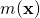, and a covariance function or kernel,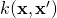. Choosing the forms of these lets us control the properties of the functions we can sample from the GP.

(1)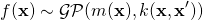When doing supervised learning (our eventual goal), we have some training data that consists of input features,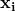, and associated outputs,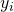, which I’ll assume to be scalar. We use this information to find a function that can take a new input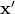and accurately predict the corresponding output,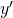. For Gaussian processes, this means we need to find mean and covariance functions which give us a GP that describes functions with properties we want (e.g. smoothness, periodicity) and can adequately fit our data.

This sounds really neat, but the idea of defining a distribution from which you can sample functions is something I have a hard time wrapping my mind around. Surprisingly this is actually fairly simple to do.

## Clojure Preliminaries

First, let’s set up our Clojure environment. For what follows, you’ll only need these dependencies in your project.clj file.

[net.mikera/core.matrix "0.36.1"] [net.mikera/vectorz-clj "0.29.0"] [yieldbot/vizard "0.1.0"]

The library core.matrix is what we’ll use for matrix and vector operations and some linear algebra. The default implementation of core.matrix uses pure Clojure data structures, which is nice, but not the best thing for efficient numerical computations. It also doesn’t support some of the numerical operations we need. Fortunately, the library defines a set of protocols that can be used to implement core.matrix functions more efficiently using your favorite Java matrix library. We will use an existing implementation called vectorz, which is our second dependency. The final dependency is our own little plotting library, vizard, because making pretty pictures is half the fun.

Now we can start up a REPL and execute:

(require '[clojure.core.matrix :as m]
'[clojure.core.matrix.protocols :as mp]
'[vizard [core :refer :all] [plot :as plot]])
(import '[java.util Random])


We’ll also need to run this:

(m/set-current-implementation :vectorz)

to make sure core.matrix uses the correct implementation.

## Sampling from a Multivariate Gaussian Distribution

When I started learning about GPs I was initially focused on the notion of a distribution that describes functions, but I realized this didn’t mean much to me when I wanted to actually
calculate things. There was another definition in GPML that was initially quite mysterious to me which defined one as a collection of random variables where any finite number of them are jointly Gaussian. This didn’t sound nearly as sexy or evocative when I first read it, but after going through the process of sampling from a Gaussian process, it became very clear how descriptive and useful this definition was.

When we sample a function from GP, we’re actually sampling values of that function at points in our input space. Perhaps that should have been obvious from the start, but this realization made everything very clear. For a single input point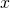, we sample the value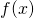. This is like sampling from a Gaussian distribution with mean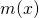and variance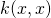. The values of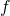at a number of points are jointly Gaussian, so sampling values offor a set of points,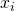, is like sampling from a multivariate Gaussian distribution with means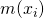and covariance matrix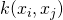. Understanding this simple point made the second definition appear much more natural.

Forgetting Gaussian processes for a moment, let’s just take a Gaussian distribution with mean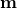and covariance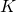and write some code to sample a vector from it.

(2)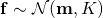We first generate a vector of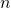independent samples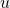from the Gaussian distribution,

(3)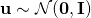which we can do with the function:

(defn sample-gaussian [n]
(let [rng (Random.)]
(repeatedly n #(.nextGaussian rng))))


Then we can transform these into samples from the desired multivariate Gaussian using:

(4)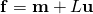where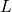is the lower triangular matrix from the Cholesky decomposition of the covariance matrix:

(5)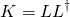and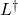is the adjoint of.

The Cholesky decomposition is one of the reasons we need a matrix library. Here’s a function that takes a mean vector and covariance matrix, decomposes the covariance, generates some Gaussian samples, and uses the transformation above to convert them into a sample with the mean and covariance we want.

(defn sample-multivariate-gaussian [mean cov]
(let [n (count mean)
e (m/scale (m/identity-matrix n) 1e-8)
L (:L (mp/cholesky (m/add (m/matrix cov) e)
{:results [:L]}))
u (m/matrix (sample-gaussian n))
(m/mmul L u))]
(m/to-nested-vectors samples)))


There’s an extra little step in there where I create an identity matrix and scale it by a small number. Adding this to the covariance matrix before doing the Cholesky decomposition helps stabilize that computation. If you find the Cholesky function returning nil, the decomposition is failing and you might have to try a slightly larger scale factor.

## Sampling Functions from a GP

Now we can sample from a Gaussian process. We begin with a GP where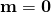and the covariance function is the squared exponential:

(6)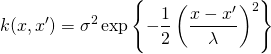In most examples I’ve seen, GP priors are chosen with mean functions that are zero, often accompanied by the phrase “without loss of generality”, which is one of my least favorite things to read because it’s rarely obvious to me why that’s the case. In this case, the mean zero prior is fine because we can always standardize the inputs to have mean of zero and variance of one. (My references to “priors” here and in the rest of this post are an indication of the Bayesian approach we’ll be taking in future posts by combining GPs like this with data to get posterior process that’s the real object of interest.) The choice of the kernel function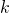, however, isn’t something that can be glossed over since it’s critical for characterizing the type of functions we want the GP to prefer. This particular choice is biased towards very smooth functions. Here are some input points at which we will sample values of:

(def test-xs (range -5 5 0.03))


and a mean vector and covariance matrix

(defn squared-exponential [sigma2 lambda x y]
(* sigma2 (Math/exp (* -0.5 (Math/pow (/ (- x y) lambda) 2)))))
(defn covariance-mat [f xs ys]
(let [rows (count xs)
cols (count ys)]
(partition cols
(for [i (range rows) j (range cols)]
(f (nth xs i) (nth ys j))))))
(defn sq-exp-cov [s2 l xs ys]
(covariance-mat (partial squared-exponential s2 l) xs ys))
(def prior-mean (repeat (count test-xs) 0.0))
(def prior-cov (sq-exp-cov 1 1 test-xs test-xs))


The variance,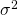, and the length scale,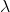, have both been set to 1.0 and we will worry about these hyperparameters in another post. Executing

(sample-multivariate-gaussian prior-mean prior-cov)


will give us a sample of the GP.

Now we can plot some examples with vizard. First, we collect our data in a format that vizard can use:

(defn vizard-pts [xs ys col]
(map (fn [x y] {:x x :y y :col col}) xs ys))
(defn line-data [xs mean cov num-samples label]
(flatten
(conj
(for [i (range num-samples)]
(vizard-pts xs
(sample-multivariate-gaussian mean cov)
(str label " sample " i)))
(vizard-pts xs mean (str label " mean")))))


The diagonal elements of the covariance matrix give us the variance at each input point which we can use to plot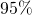confidence bands. This will be a little boring in this case because the variance is 1.0 everywhere. In subsequent posts this will be more interesting as we incorporate observational data into the covariance matrix.

(defn conf-data [xs mean cov]
(let [std-dev (map #(Math/sqrt %) (m/diagonal cov))]
(map (fn [x m s]
{:x x :y (+ m (* 2 s)) :y2 (- m (* 2 s))})
xs mean std-dev)))


And finally we can start the plot server

(start-plot-server!)


and display our results with this function:

(defn plot-gp [line-data conf-data]
(plot! (-> (plot/vizard {:mark-type :line
:color "category20b"}
line-data)
(assoc-in [:data 1]
{:name :confidence
:values conf-data})
(assoc-in [:marks 1]
{:type :area
:from {:data :confidence}
:properties
{:enter
{:x {:scale "x" :field :x}
:y {:scale "y" :field :y}
:y2 {:scale "y" :field :y2}
:interpolate {:value :monotone}
:fill {:value "#666"}}
:update {:fillOpacity {:value 0.25}}}}))))


(The basic vizard function is sufficient to graph our functions, but I’m assoc-ing in some extra data and marks into the Vega spec to show confidence bands.)

(let [prior-data (line-data test-xs prior-mean prior-cov 3 "prior")
prior-conf-data (conf-data test-xs prior-mean prior-cov)]
(plot-gp prior-data prior-conf-data))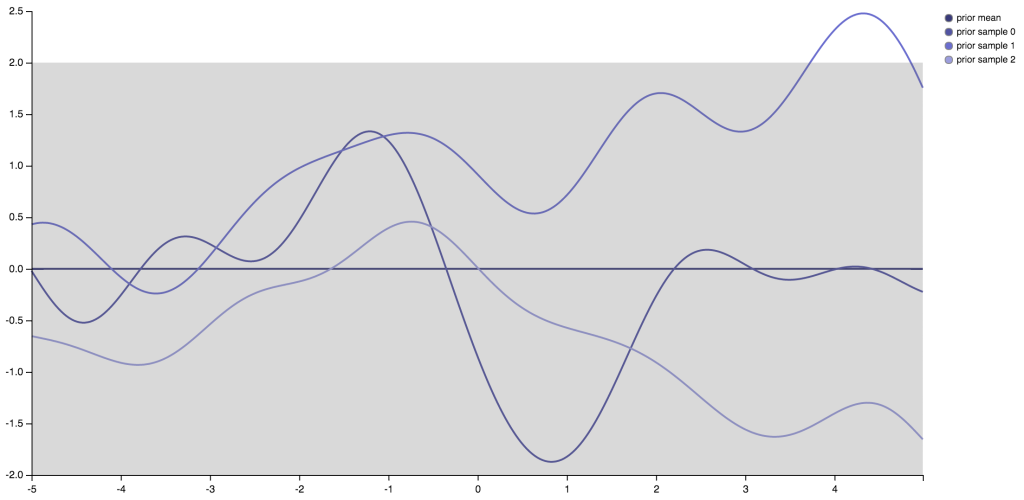The mean function for the GP is zero, but the function will be nonzero at almost all inputs.sampled at some point could be anything, though it is likely to be closer to zero then far away. At any fixed point, samples ofare just samples from a Gaussian distribution with mean zero and variance.

It’s still a bit magical to me that we get these nice smooth functions out of this process, but that’s where the covariance function comes in. When we sample values ofat multiple input points,, the covariance function makes sure that values ofsampled at nearby points are similar. In this context, “nearby” is defined by the length scaleand we’ll see how that works near the end of this post.

Our choice of the squared exponential function defines a particular form of similarity between differents, but we can choose other kernels to select functions with additional properties. For example, if we were doing time series forecasting and needed functions to reflect weekly seasonal variations in the data, we could use a covariance function that treats points 7 days apart as similar.

Let’s just try a small modification to the covariance function and replace the square in the exponential with an absolute value and see what happens.

(7)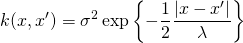(defn abs-exponential [sigma2 lambda x y]
(* sigma2 (Math/exp (* -0.5 (Math/abs (/ (- x y) lambda))))))
(defn abs-exp-cov [s2 l xs ys]
(covariance-mat (partial abs-exponential s2 l) xs ys))
(def abs-prior-cov (abs-exp-cov 1 1 test-xs test-xs))
(let [prior-data (line-data test-xs prior-mean abs-prior-cov 3 "prior")
prior-conf-data (conf-data test-xs prior-mean abs-prior-cov)]
(plot-gp prior-data prior-conf-data))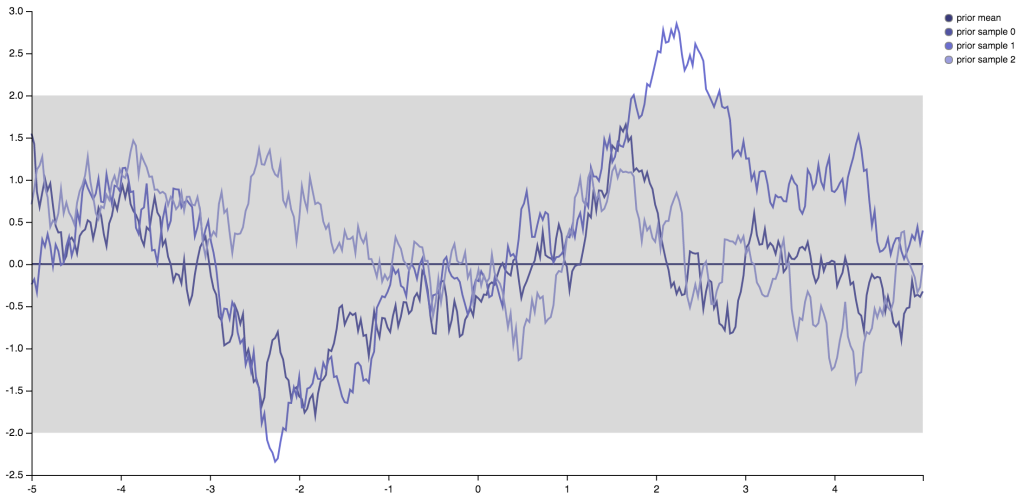It turns out that this little change takes us from a GP that prefers infinitely differentiable functions to one that gives us functions that are merely continuous, but not differentiable. I think that’s pretty neat. Both of these kernels are members of the Matérn class of covariance functions. You can use functions from this class to tune the smoothness of the functions sampled from your Gaussian process.

## Closing Remarks

I don’t know if sampling from a GP is ever useful in practice, but going through this exercise of producing them was at least educational and entertaining for me. In an upcoming post I will take the next step and incorporate observations to find a posterior Gaussian process and solve a simple regression problem.